Next: Magnetohydrodynamic Fluids Up: Waves in Cold Plasmas Previous: Ray Tracing

# Radio Wave Propagation Through the Ionosphere

To a first approximation, the Earth's ionosphere consists of an unmagnetized, horizontally stratified, partially ionized gas. The dispersion relation for the electromagnetic plasma wave takes the form [see Eq. (546)]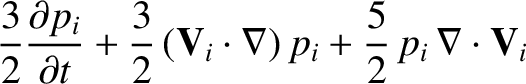(661)

where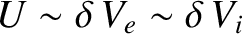(662)

Here,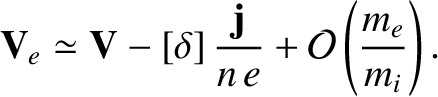is the density of free electrons in the ionosphere, and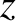is a coordinate which measures height above the surface of the Earth. (N.B., The curvature of the Earth is neglected in the following analysis.)

Now,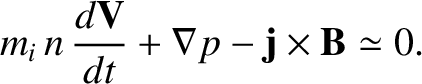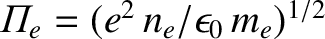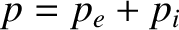(663)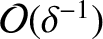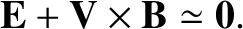(664)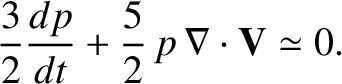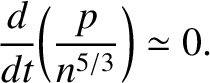(665)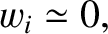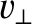(666)

Thus, the ray equations, (658)-(660), yield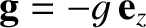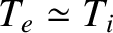(667)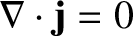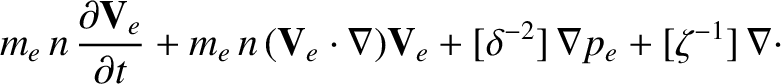(668)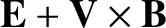(669)

Note that the frequency of a radio pulse does not change as it propagates through the ionosphere, provided that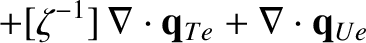does not vary in time. It is clear, from Eqs. (667)-(669), and the fact that, that a radio pulse which starts off at ground level propagating in the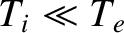-plane, say, will continue to propagate in this plane.

For pulse propagation in the-plane, we have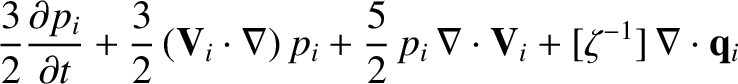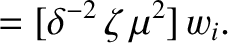(670)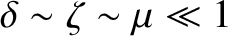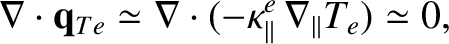(671)(672)

The dispersion relation (661) yields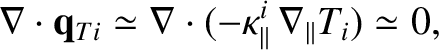(673)

where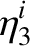is the refractive index.

We assume that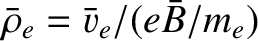at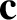, which is equivalent to the reasonable assumption that the atmosphere is non-ionized at ground level. It follows from Eq. (672) that(674)

where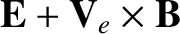is the sine of the angle of incidence of the pulse, with respect to the vertical axis, at ground level. Equations (673) and (674) yield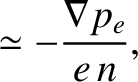(675)

According to Eq. (671), the plus sign corresponds to the upward trajectory of the pulse, whereas the minus sign corresponds to the downward trajectory. Finally, Eqs. (670), (671), (674), and (675) yield the equations of motion of the pulse:(676)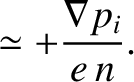(677)

The pulse attains its maximum altitude,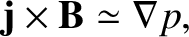, when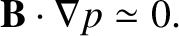(678)

The total distance traveled by the pulse (i.e., the distance from its launch point to the point where it intersects the Earth's surface again) is(679)

In the limit in which the radio pulse is launched vertically (i.e.,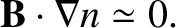) into the ionosphere, the turning point condition (678) reduces to that characteristic of a cutoff (i.e.,). The WKB turning point described in Eq. (678) is a generalization of the conventional turning point, which occurs whenchanges sign. Here,changes sign, whilstand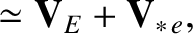are constrained by symmetry (i.e.,is constant, and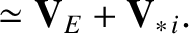is zero).

According to Eqs. (667)-(669) and (673), the equation of motion of the pulse can also be written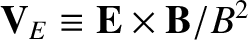(680)

It follows that the trajectory of the pulse is the same as that of a particle moving in the gravitational potential. Thus, if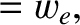decreases linearly with increasing height above the ground [which is the case ifincreases linearly with] then the trajectory of the pulse is a parabola.Next: Magnetohydrodynamic Fluids Up: Waves in Cold Plasmas Previous: Ray Tracing
Richard Fitzpatrick 2011-03-31